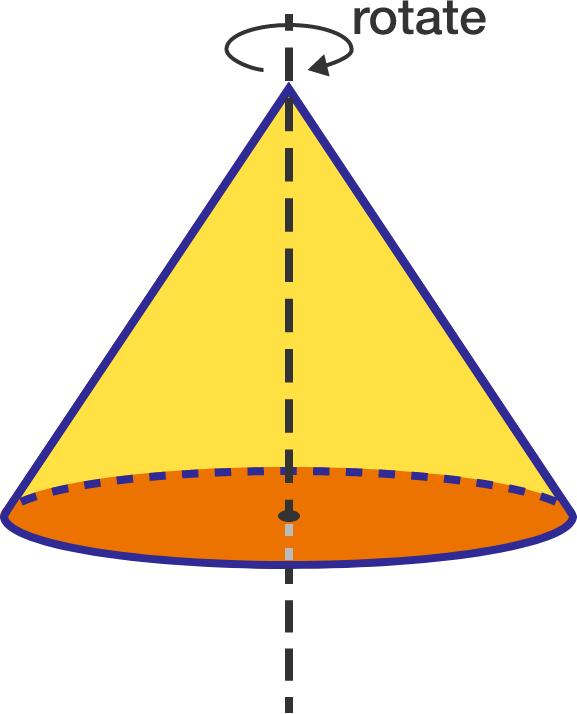# Frictional torqueA uniform solid right-circular cone of mass $M$ and radius $R$ is kept on a rough horizontal floor (coefficient of friction $\mu$) on its circular base. It is spun with initial angular velocity $\omega_{0}$ about its symmetry axis. Neglecting toppling effects (if any), find the time after which it stops spinning.


Details and Assumptions:

• Take the moment of inertia of the cone about its symmetry axis as $I$.

×